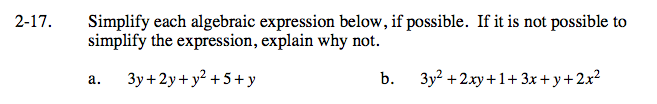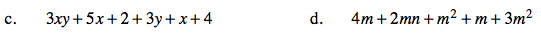### Home > CAAC > Chapter 2 > Lesson 2.1.2 > Problem2-17

2-17.
1. Simplify each algebraic expression below, if possible. If it is not possible to simplify the expression, explain why not. Homework Help ✎

1. 3y + 2y + y2 + 5 + y

2. 3y2 + 2xy + 1 + 3x + y + 2x2

3. 3xy + 5x + 2 + 3y + x + 4

4. 4m + 2mn + m2 + m + 3m2Combine like terms.

3y + 2y + y + y2 + 5

6y + y2 + 5

Arrange from the highest to the lowest power.

y2 + 6y + 5

Is there any way to combine terms?
Remember, you can only add terms if they have the exact same variable.Follow steps taken in part (a).

Follow the steps taken in part (a).

4m2 + 5m + 2mn